2010-12-09 14:39:00 wqvbjhc 阅读数 5167
• ###### 机器学习之概率与统计推断

本课程讲解机器学习算法所需概率和统计推断知识。概率部分包括概率公理及推论、条件概率、贝叶斯公式、随机变量及其概率函数（CDF／pdf）、常用概率分布及其均值、方差；统计推断部分包括大数定律和中心极限定理、极大似然估计、贝叶斯估计，估计的评价、偏差-方差平衡。课程还会讲解假设检验的基本概念。

20328 人正在学习 去看看 AI100讲师

# 灰度图像均值平滑

图像平滑用于去除图像中的噪声。均值平滑，就是将每个像素的灰度值用其领域的平均值代替。该算法简单，速度快，但不能完全消除椒盐噪声。
平滑模板：

 1 1 1 1 1 1 1 1 12019-05-03 17:28:20 zaishuiyifangxym 阅读数 6381
• ###### 机器学习之概率与统计推断

本课程讲解机器学习算法所需概率和统计推断知识。概率部分包括概率公理及推论、条件概率、贝叶斯公式、随机变量及其概率函数（CDF／pdf）、常用概率分布及其均值、方差；统计推断部分包括大数定律和中心极限定理、极大似然估计、贝叶斯估计，估计的评价、偏差-方差平衡。课程还会讲解假设检验的基本概念。

20328 人正在学习 去看看 AI100讲师1 图像增强——图像平滑

1.1 图像增强简介

1.2 图像平滑

2 均值滤波

3 中值滤波

4 高斯滤波

# 1 图像增强——图像平滑

## 1.1 图像增强简介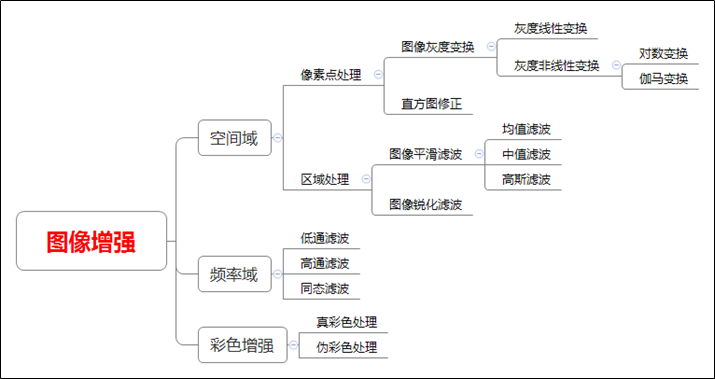## 1.2 图像平滑

# -*- coding:utf-8 -*-
import cv2
import numpy as np

# 读取图片
img_noise=img

cv2.imshow("src", img)

rows, cols, chn = img_noise.shape

# 加噪声
for i in range(5000):
x = np.random.randint(0, rows)
y = np.random.randint(0, cols)
img_noise[x, y, :] = 255

cv2.imshow("noise", img_noise)

# 等待显示
cv2.waitKey(0)
cv2.destroyAllWindows()

#保存含噪声图像
cv2.imwrite("zxp_noise.jpg", img_noise)# 2 均值滤波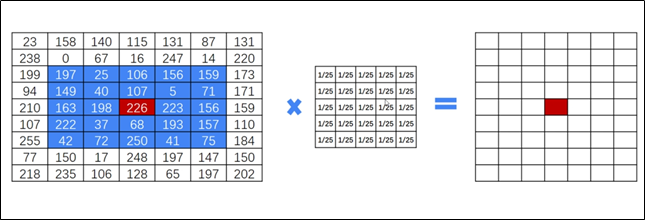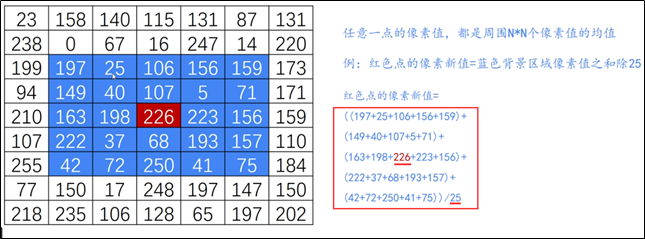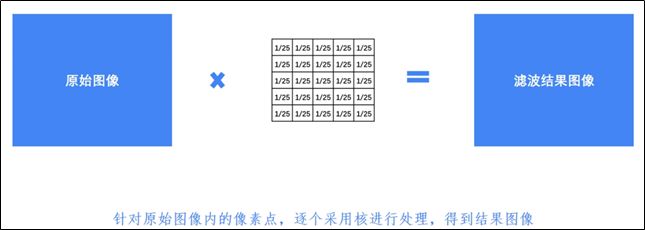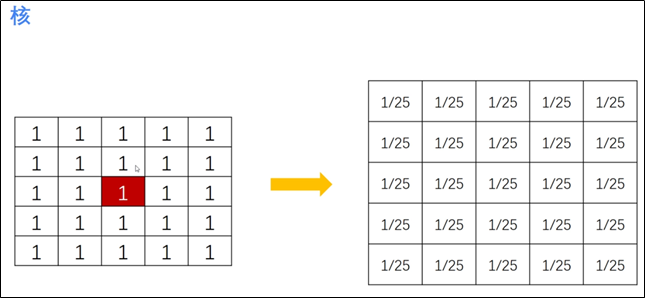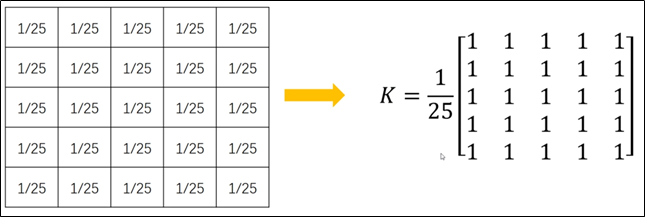Python调用OpenCV实现 均值滤波 的函数如下：

result = cv2.blur(原始图像,核大小)

（1） 核大小为 33

# encoding:utf-8
import cv2
import numpy as np
import matplotlib.pyplot as plt

# 读取图片
source = cv2.cvtColor(img, cv2.COLOR_BGR2RGB)

# 均值滤波
result = cv2.blur(source, (3, 3)) #可以更改核的大小

# 显示图形
titles = ['Source Image', 'Blur Image (3, 3)']
images = [source, result]
for i in range(2):
plt.subplot(1, 2, i + 1), plt.imshow(images[i], 'gray')
plt.title(titles[i])
plt.xticks([]), plt.yticks([])
plt.show()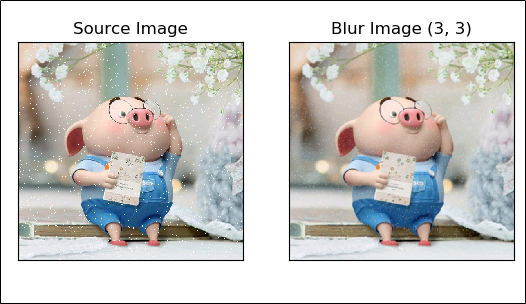（2） 核大小为 55

# encoding:utf-8
import cv2
import numpy as np
import matplotlib.pyplot as plt

# 读取图片
source = cv2.cvtColor(img, cv2.COLOR_BGR2RGB)

# 均值滤波
result = cv2.blur(source, (5, 5)) #可以更改核的大小

# 显示图形
titles = ['Source Image', 'Blur Image (5, 5)']
images = [source, result]
for i in range(2):
plt.subplot(1, 2, i + 1), plt.imshow(images[i], 'gray')
plt.title(titles[i])
plt.xticks([]), plt.yticks([])
plt.show()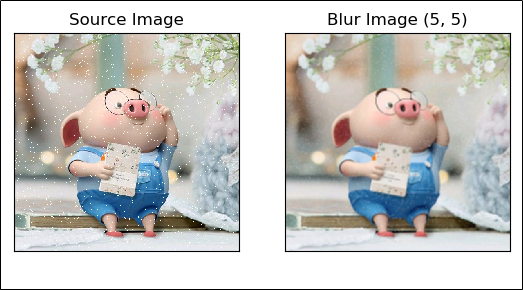（2） 核大小为 1010

# encoding:utf-8
import cv2
import numpy as np
import matplotlib.pyplot as plt

# 读取图片
source = cv2.cvtColor(img, cv2.COLOR_BGR2RGB)

# 均值滤波
result = cv2.blur(source, (10, 10)) #可以更改核的大小

# 显示图形
titles = ['Source Image', 'Blur Image (10, 10)']
images = [source, result]
for i in range(2):
plt.subplot(1, 2, i + 1), plt.imshow(images[i], 'gray')
plt.title(titles[i])
plt.xticks([]), plt.yticks([])
plt.show()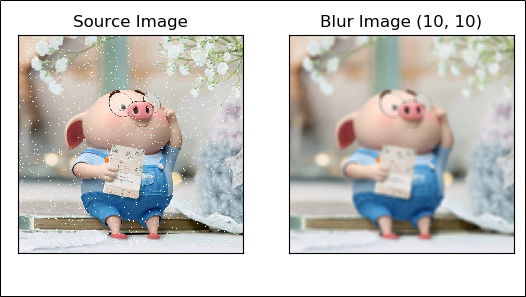1）随着核大小逐渐变大，会让图像变得更加模糊；

2）如果设置为核大小为（1，1），则结果就是原始图像。

# 3 中值滤波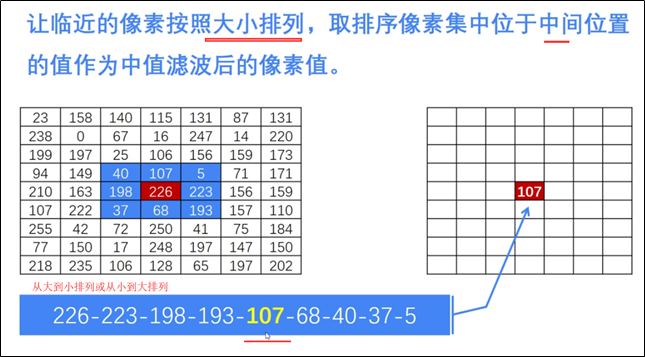Python调用OpenCV实现 中值滤波 的函数如下：

OpenCV主要调用 medianBlur() 函数实现中值滤波。图像平滑里中值滤波的效果最好。

dst = cv2.medianBlur(src, ksize)

src 表示源图像；

ksize 表示核大小。核必须是大于1的奇数，如3、5、7等。

（1）核大小为 33

# encoding:utf-8
import cv2
import numpy as np
import matplotlib.pyplot as plt

# 读取图片

# 中值滤波
result = cv2.medianBlur(img, 3)#可以更改核的大小

# 显示图像
cv2.imshow("source img", img)
cv2.imshow("medianBlur", result)

# 等待显示
cv2.waitKey(0)
cv2.destroyAllWindows()（2）核大小为 55

# encoding:utf-8
import cv2
import numpy as np
import matplotlib.pyplot as plt

# 读取图片

# 中值滤波
result = cv2.medianBlur(img, 5) #可以更改核的大小

# 显示图像
cv2.imshow("source img", img)
cv2.imshow("medianBlur", result)

# 等待显示
cv2.waitKey(0)
cv2.destroyAllWindows()（3）核大小为 77

# encoding:utf-8
import cv2
import numpy as np
import matplotlib.pyplot as plt

# 读取图片

# 中值滤波
result = cv2.medianBlur(img, 7) #可以更改核的大小

# 显示图像
cv2.imshow("source img", img)
cv2.imshow("medianBlur", result)

# 等待显示
cv2.waitKey(0)
cv2.destroyAllWindows()1）随着核大小逐渐变大，会让图像变得更加模糊；

2）核必须是大于1的奇数，如3、5、7等；

3）在代码 dst = cv2.medianBlur(src, ksize) 中 填写核大小时，只需填写一个数即可，如3、5、7等，对比均值滤波函数用法。

# 4 高斯滤波

（1）核大小为 33

（1）核大小为 55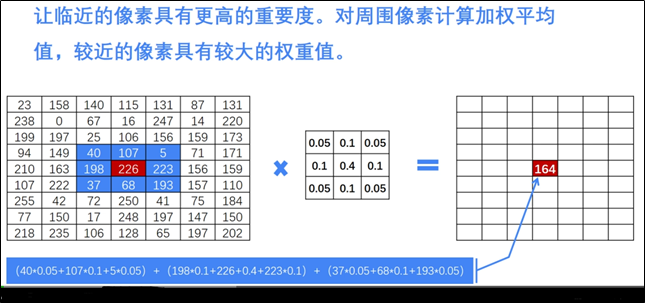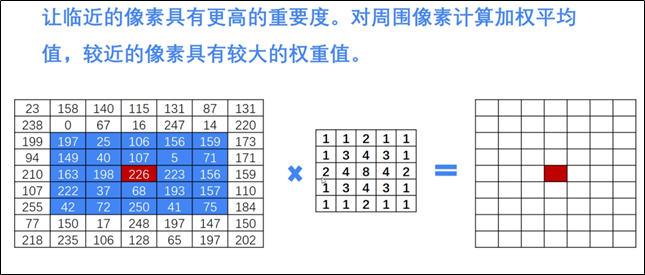Python中OpenCV主要调用 GaussianBlur() 函数，如下：

dst = cv2.GaussianBlur(src, ksize, sigmaX)

src 表示原始图像；

ksize 表示核大小；

sigmaX 表示X方向方差。

：核大小（N, N）必须是奇数，X方向方差主要控制权重。

1）核大小为 33

2）核大小为 55

（1）核大小为 33


# encoding:utf-8
import cv2
import numpy as np
import matplotlib.pyplot as plt

# 读取图片
source = cv2.cvtColor(img, cv2.COLOR_BGR2RGB)

# 高斯滤波
result = cv2.GaussianBlur(source, (3, 3), 0) #可以更改核大小

# 显示图形
titles = ['Source Image', 'GaussianBlur Image (3, 3)']
images = [source, result]
for i in range(2):
plt.subplot(1, 2, i + 1), plt.imshow(images[i], 'gray')
plt.title(titles[i])
plt.xticks([]), plt.yticks([])
plt.show()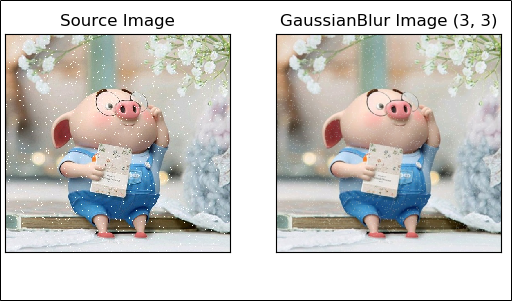（2）核大小为 55

# encoding:utf-8
import cv2
import numpy as np
import matplotlib.pyplot as plt

# 读取图片
source = cv2.cvtColor(img, cv2.COLOR_BGR2RGB)

# 高斯滤波
result = cv2.GaussianBlur(source, (5, 5), 0) #可以更改核大小

# 显示图形
titles = ['Source Image', 'GaussianBlur Image (5, 5)']
images = [source, result]
for i in range(2):
plt.subplot(1, 2, i + 1), plt.imshow(images[i], 'gray')
plt.title(titles[i])
plt.xticks([]), plt.yticks([])
plt.show()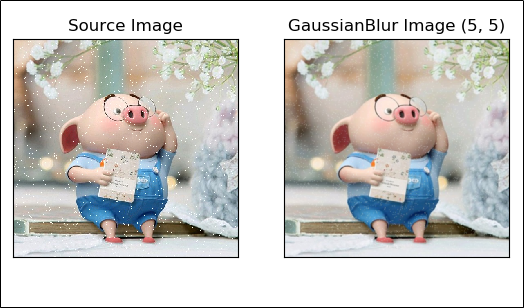1）随着核大小逐渐变大，会让图像变得更加模糊；

2）核大小（N, N）必须是大于1的奇数，如3、5、7等；

# 参考资料

2018-03-07 23:21:25 u012421852 阅读数 514
• ###### 机器学习之概率与统计推断

本课程讲解机器学习算法所需概率和统计推断知识。概率部分包括概率公理及推论、条件概率、贝叶斯公式、随机变量及其概率函数（CDF／pdf）、常用概率分布及其均值、方差；统计推断部分包括大数定律和中心极限定理、极大似然估计、贝叶斯估计，估计的评价、偏差-方差平衡。课程还会讲解假设检验的基本概念。

20328 人正在学习 去看看 AI100讲师
20180307站位

2019-09-18 16:16:40 caimouse 阅读数 79
• ###### 机器学习之概率与统计推断

本课程讲解机器学习算法所需概率和统计推断知识。概率部分包括概率公理及推论、条件概率、贝叶斯公式、随机变量及其概率函数（CDF／pdf）、常用概率分布及其均值、方差；统计推断部分包括大数定律和中心极限定理、极大似然估计、贝叶斯估计，估计的评价、偏差-方差平衡。课程还会讲解假设检验的基本概念。

20328 人正在学习 去看看 AI100讲师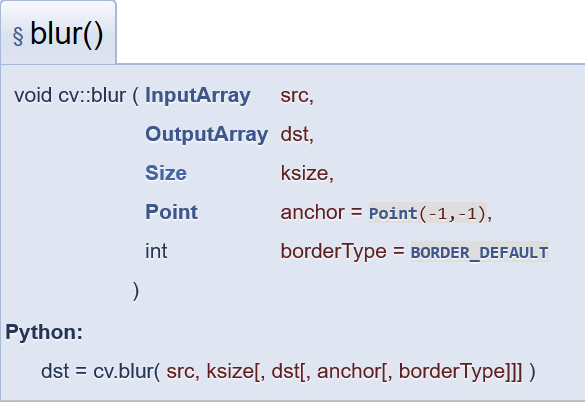src 输入图像

dst 输出图像

ksize 平滑窗口的大小

anchor 锚点，如果宽、高均为奇数，则Point(-1,-1)代表中心点

borderType 边界扩充类型

#python 3.7.4,opencv4.1
#蔡军生 https://blog.csdn.net/caimouse/article/details/51749579
#
import cv2
import numpy as np

#图片的路径
imgname = "gauss1.jpg"

#读取图片

#图片的高度和宽度
h,w = image.shape[:2]
print('imagesize={}-{}'.format(w,h))

#显示原图
cv2.imshow("Image",image)

#平滑
out = cv2.blur(image,(5,5))
out = out.astype(np.uint8)
cv2.imshow("out",out)

cv2.waitKey(0)
cv2.destroyAllWindows()https://blog.csdn.net/caimouse/article/details/51749579

2013-04-10 14:51:37 mlkiller 阅读数 4237
• ###### 机器学习之概率与统计推断

本课程讲解机器学习算法所需概率和统计推断知识。概率部分包括概率公理及推论、条件概率、贝叶斯公式、随机变量及其概率函数（CDF／pdf）、常用概率分布及其均值、方差；统计推断部分包括大数定律和中心极限定理、极大似然估计、贝叶斯估计，估计的评价、偏差-方差平衡。课程还会讲解假设检验的基本概念。

20328 人正在学习 去看看 AI100讲师

# 一含义

模糊（平滑）是一种常用的图片处理方式，它的作用可以用来降低噪声，还有其他用途

看一下opencv 里面的公式g(i,j)是目标坐标的像素值， f(i+k,j+l)是k,l这些地方的像素值， h(k,l)是 kernel,  我不知道怎么去准确翻译它的意义，它是过滤器的系数。

简单的按照我的思路去理解，就是一个权值，模糊的含义是将所有的像素按照一定的权值进行运算，得到一个比较均衡的结果。

# 三 算法

1 均值模糊
均值模糊很简单就是周边所有的影响都是1，求平均值即可2 高斯模糊# 四均值模糊的代码和效果

先放上均值模糊的代码
void boxblur(Mat input ,Mat &out, int x, int y)
{
// accept only char type matrices
CV_Assert(input.depth() != sizeof(uchar));

out.create(input.size(),input.type());

int nChannels = input.channels();
int nRows = input.rows;
int nCols = input.cols;

int size = x * y;
float kernel = 1.0/size;

int i,j;
uchar* p;
uchar* q;
uchar R,G,B;

for( i = x; i < nRows - x; ++i)
{
q = out.ptr<uchar>(i);
for ( j = y; j < nCols - y; ++j)
{
float sumR = 0;
float sumG = 0;
float sumB = 0;
for (int k =0; k<x;k++)
{
p = input.ptr<uchar>(i-x+k);
for(int l = 0; l < y;l++)
{
sumB += input.at<uchar>(i - x + k,(j + l - y)*nChannels) * kernel;//p[(l + j -y)*nChannels ] * kernel;
sumG += input.at<uchar>(i - x + k,(j + l - y)*nChannels + 1) * kernel;//p[(l + j -y)*nChannels + 1] * kernel;
sumR += input.at<uchar>(i - x + k,(j + l - y)*nChannels + 2) * kernel;//p[(l + j -y)*nChannels + 2] * kernel;
}
}
q[j*nChannels] = sumB;
q[j*nChannels+1] = sumG;
q[j*nChannels+2] = sumR;
}
}

}原始 opencv 本文# 五高斯模糊的代码和效果

void gaussblur(Mat input ,Mat &out, int x, int y)
{
float sigma = 1.5;
Mat kernel;
float pi = 3.1415926;

kernel.create(x ,y ,CV_32F);

float mx = x/2.0;
float my = y/2.0;


       //这里有问题，后面做修正。
for (int i =0; i< x;i++)
{
for (int j =0; j<y;j++)
{
kernel.at<float>(i,j) = exp(-1 * ((i - mx) * (i - mx) +(j - my) * (j-my) )/( 2 * sigma * sigma))/(2 * pi * sigma *sigma) ;
}
}


int nChannels = input.channels();
int nRows = input.rows;
int nCols = input.cols;

out.create(input.size(),input.type());
uchar* p;
uchar* q;
float* s;

for(int  i = x; i < nRows - x; ++i)
{
q = out.ptr<uchar>(i);
for (int j = y; j < nCols - y; ++j)
{
float sumR = 0;
float sumG = 0;
float sumB = 0;
for (int k =0; k<x;k++)
{
p = input.ptr<uchar>(i-x+k);
s = kernel.ptr<float>(k);
for(int l = 0; l < y;l++)
{
sumB += p[(l + j -y)*nChannels ] * s[l];//input.at<uchar>(i - x + k,(j + l - y)*nChannels) * kernel;//
sumG += p[(l + j -y)*nChannels + 1] *s[l];//input.at<uchar>(i - x + k,(j + l - y)*nChannels + 1) * kernel;//
sumR += p[(l + j -y)*nChannels + 2] * s[l];//input.at<uchar>(i - x + k,(j + l - y)*nChannels + 2) * kernel;
}
}
q[j*nChannels] = sumB;
q[j*nChannels+1] = sumG;
q[j*nChannels+2] = sumR;
}
}

}原始 opencv 本文float sum = 0;
for (int i =0; i< x;i++)
{
for (int j =0; j<y;j++)
{
sum+= kernel.at<float>(i,j) = exp(-1 * ((i - mx) * (i - mx) +(j - my) * (j-my) )/( 2 * sigma * sigma))/(2 * pi * sigma *sigma) ;
}
}
for (int i =0; i< x;i++)
{
for (int j =0; j<y;j++)
{
kernel.at<float>(i,j) = kernel.at<float>(i,j)/ sum ;
}
}

sigma = 0.3*((ksize-1)*0.5 - 1) + 0.8 .